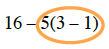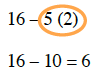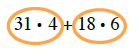### Home > MC2 > Chapter 2 > Lesson 2.3.6 > Problem2-152

2-152.

Simplify each expression. You may want to refer to the Math Notes box for help.

1. $16-5(3-1)$1. $31 · 4 + 18 · 6$$124 + 108 = 232$

1. $3(2^2)-8 · 1$

Refer to the Math Notes box in Lesson 2.3.6 and parts (a) and (c).

1. $4 · 5-2^2 + 3(5-4)$

Refer to the Math Notes box in Lesson 2.3.6 and parts (a) and (c).